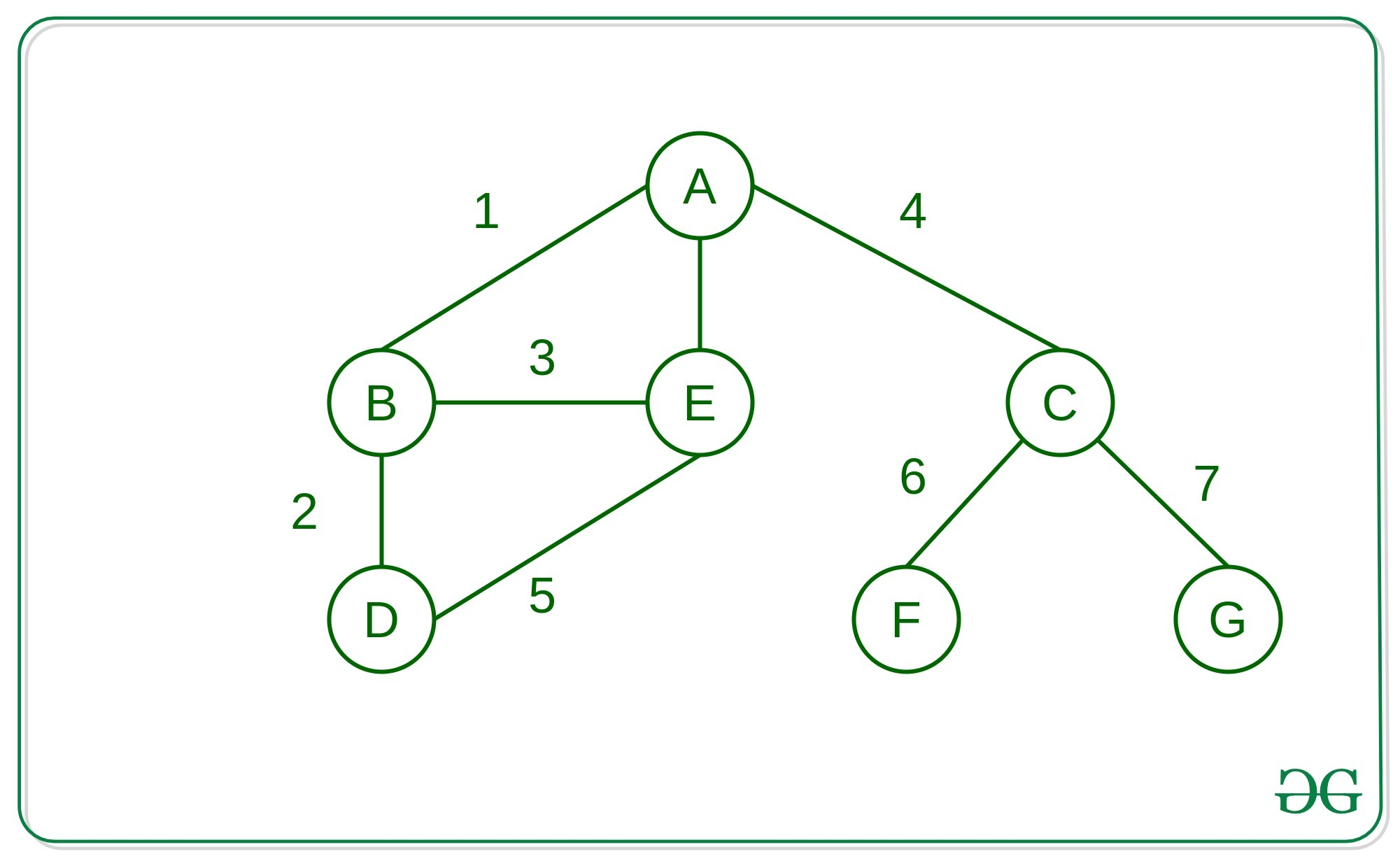# Building an undirected graph and finding shortest path using Dictionaries in Python

• Difficulty Level : Hard
• Last Updated : 15 Dec, 2021

Prerequisites:

In this article, we will be looking at how to build an undirected graph and then find the shortest path between two nodes/vertex of that graph easily using dictionaries in Python Language.

### Building a Graph using DictionariesApproach: The idea is to store the adjacency list into the dictionaries, which helps to store the graph in any format not only in the form of the integers. Here we have used characters as a reference on those places any custom objects can also be used.

Below is the implementation of the above approach:

## Python3

 `# Python3 implementation to build a ` `# graph using Dictionaries`   `from` `collections ``import` `defaultdict`   `# Function to build the graph` `def` `build_graph():` `    ``edges ``=` `[` `        ``[``"A"``, ``"B"``], [``"A"``, ``"E"``], ` `        ``[``"A"``, ``"C"``], [``"B"``, ``"D"``],` `        ``[``"B"``, ``"E"``], [``"C"``, ``"F"``],` `        ``[``"C"``, ``"G"``], [``"D"``, ``"E"``]` `    ``]` `    ``graph ``=` `defaultdict(``list``)` `    `  `    ``# Loop to iterate over every ` `    ``# edge of the graph` `    ``for` `edge ``in` `edges:` `        ``a, b ``=` `edge[``0``], edge[``1``]` `        `  `        ``# Creating the graph ` `        ``# as adjacency list` `        ``graph[a].append(b)` `        ``graph[b].append(a)` `    ``return` `graph`   `if` `__name__ ``=``=` `"__main__"``:` `    ``graph ``=` `build_graph()` `    `  `    ``print``(graph)`

Output:

```{
'G': ['C'],
'F': ['C'],
'E': ['A', 'B', 'D'],
'A': ['B', 'E', 'C'],
'B': ['A', 'D', 'E'],
'D': ['B', 'E'],
'C': ['A', 'F', 'G']
}```

### Shortest Path between two nodes of graph

Approach: The idea is to use queue and visit every adjacent node of the starting nodes that traverses the graph in Breadth-First Search manner to find the shortest path between two nodes of the graph.

Below is the implementation of the above approach:

## Python3

 `# Python implementation to find the ` `# shortest path in the graph using ` `# dictionaries `   `# Function to find the shortest` `# path between two nodes of a graph` `def` `BFS_SP(graph, start, goal):` `    ``explored ``=` `[]` `    `  `    ``# Queue for traversing the ` `    ``# graph in the BFS` `    ``queue ``=` `[[start]]` `    `  `    ``# If the desired node is ` `    ``# reached` `    ``if` `start ``=``=` `goal:` `        ``print``(``"Same Node"``)` `        ``return` `    `  `    ``# Loop to traverse the graph ` `    ``# with the help of the queue` `    ``while` `queue:` `        ``path ``=` `queue.pop(``0``)` `        ``node ``=` `path[``-``1``]` `        `  `        ``# Condition to check if the` `        ``# current node is not visited` `        ``if` `node ``not` `in` `explored:` `            ``neighbours ``=` `graph[node]` `            `  `            ``# Loop to iterate over the ` `            ``# neighbours of the node` `            ``for` `neighbour ``in` `neighbours:` `                ``new_path ``=` `list``(path)` `                ``new_path.append(neighbour)` `                ``queue.append(new_path)` `                `  `                ``# Condition to check if the ` `                ``# neighbour node is the goal` `                ``if` `neighbour ``=``=` `goal:` `                    ``print``(``"Shortest path = "``, ``*``new_path)` `                    ``return` `            ``explored.append(node)`   `    ``# Condition when the nodes ` `    ``# are not connected` `    ``print``(``"So sorry, but a connecting"``\` `                ``"path doesn't exist :("``)` `    ``return`   `# Driver Code` `if` `__name__ ``=``=` `"__main__"``:` `    `  `    ``# Graph using dictionaries` `    ``graph ``=` `{``'A'``: [``'B'``, ``'E'``, ``'C'``],` `            ``'B'``: [``'A'``, ``'D'``, ``'E'``],` `            ``'C'``: [``'A'``, ``'F'``, ``'G'``],` `            ``'D'``: [``'B'``, ``'E'``],` `            ``'E'``: [``'A'``, ``'B'``, ``'D'``],` `            ``'F'``: [``'C'``],` `            ``'G'``: [``'C'``]}` `    `  `    ``# Function Call` `    ``BFS_SP(graph, ``'A'``, ``'D'``)`

Output:

`Shortest path =  A B D`

My Personal Notes arrow_drop_up
Recommended Articles
Page :